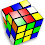## Friday, April 04, 2008

### Hitting the Target Puzzle

Here's a quick puzzle. In the attached image, a circle is inscribed in a square which is inscribed in another circle.

Of the outside yellow ring, or the inside purple circle, which has the bigger area, and why?

1.Imagine the square root of 2.

2.Well, the inner circle has an area of pi*radius**2.

The radius of the larger circle is a function of the hypotenuse of the square. The hypotenuse(RADIUS**2) of the square is (2*radius)**2 + (2*radius)**2

So, the area of the outer circle is pi*8*radius**2. Subtract the inner circle area and you get pi*7*radius**2 for the outer area, which is greater than the area of the inner circle.

3.Don, double-check your math. The yellow ring does NOT have 7 times the area of the magenta circle.

4.Yes, I left an extra '2' in the equation. Shame.

The areas are equal.

5.Well done, all!

Solution diagram

The key is the square. Looking at the two circles, the ratio of their radii will be sqrt(2) : 1 because they are the hypotenuse and leg of a 45-45-90 triangle).

The circles will therefore have areas in the ratio of 2 : 1. When you subtract the inner circle to leave just the ring, you have two areas that are equal.

For NPR puzzle posts, don't post the answer or any hints that could lead to the answer before the deadline (usually Thursday at 3pm ET). If you know the answer, submit it to NPR, but don't give it away here.

You may provide indirect hints to the answer to show you know it, but make sure they don't assist with solving. You can openly discuss your hints and the answer after the deadline. Thank you.